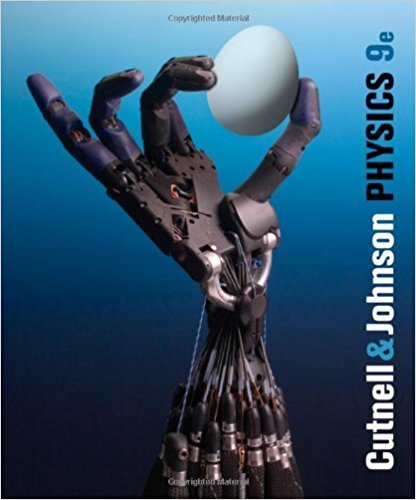×
×

# Solutions for Chapter 3: Kinematics in Two Dimensions## Full solutions for Physics, | 9th Edition

ISBN: 9780470879528Solutions for Chapter 3: Kinematics in Two Dimensions

Solutions for Chapter 3
4 5 0 405 Reviews
16
4
##### ISBN: 9780470879528

This expansive textbook survival guide covers the following chapters and their solutions. Physics, was written by and is associated to the ISBN: 9780470879528. Since 112 problems in chapter 3: Kinematics in Two Dimensions have been answered, more than 73351 students have viewed full step-by-step solutions from this chapter. This textbook survival guide was created for the textbook: Physics,, edition: 9. Chapter 3: Kinematics in Two Dimensions includes 112 full step-by-step solutions.

Key Physics Terms and definitions covered in this textbook
• //

parallel

• any symbol

average (indicated by a bar over a symbol—e.g., v¯ is average velocity)

• °C

Celsius degree

• °F

Fahrenheit degree

×